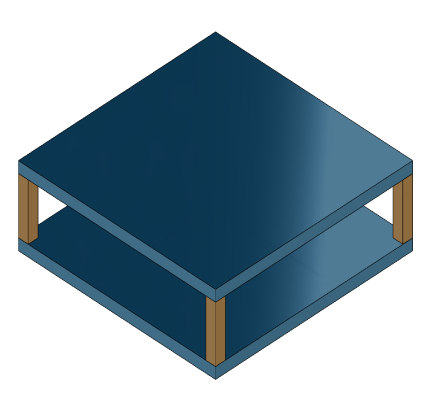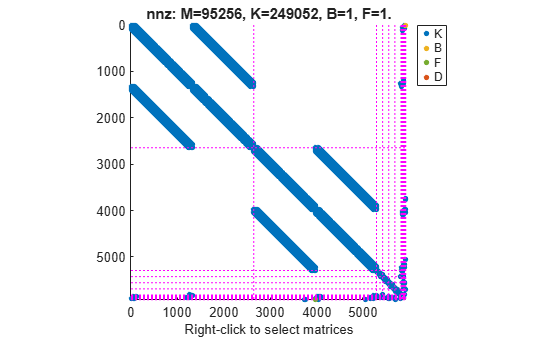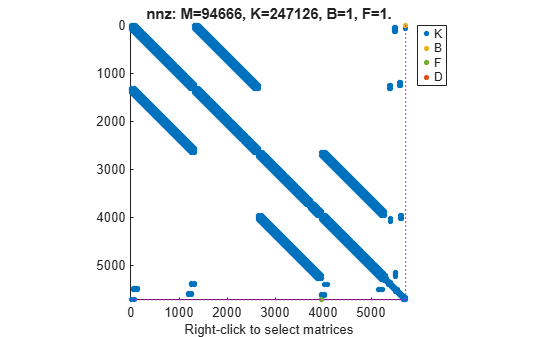# interface

Specify physical connections between components of `mechss` model

## Syntax

``sysCon = interface(sys,C1,IC1,C2,IC2)``
``sysCon = interface(sys,C,IC)``
``sysCon = interface(___,KI,CI)``
``sysCon = interface(___,method)``

## Description

example

````sysCon = interface(sys,C1,IC1,C2,IC2)` specifies physical couplings between components `C1` and `C2` in the second-order sparse model `sys`. `IC1` and `IC2` contain the indices of the coupled degrees of freedom (DOFs) relative to the DOFs of `C1` and `C2`. The physical interface is assumed rigid and satisfies the standard consistency and equilibrium conditions. `sysCon` is the resultant model with the specified physical connections. Use `showStateInfo` to get the list of all available components of `sys`.```

example

````sysCon = interface(sys,C,IC)` specifies that component `C` interfaces with the ground. Connecting the degrees of freedom q of `C` to the ground amounts to the zero displacement constraint `q(IC) = 0`.```
````sysCon = interface(___,KI,CI)` further specifies the stiffness `KI` and damping `CI` for nonrigid interfaces.```
````sysCon = interface(___,method)` specifies the assembly method. By default, `method` = `'dual'` and the function uses the dual-assembly method of physical coupling. Set `method` = `'primal'` to use the primal-assembly method of physical coupling. For more information, see Algorithms.```

## Examples

collapse all

For this example, consider a structural model that consists of two square plates connected with pillars at each vertex as depicted in the figure below. The lower plate is attached rigidly to the ground while the pillars are attached rigidly to each vertex of the square plate.Load the finite element model matrices contained in `platePillarModel.mat` and create the sparse second-order model representing the above system.

```load('platePillarModel.mat') model = ... mechss(M1,[],K1,B1,F1,'Name','Plate1') + ... mechss(M2,[],K2,B2,F2,'Name','Plate2') + ... mechss(Mp,[],Kp,Bp,Fp,'Name','Pillar3') + ... mechss(Mp,[],Kp,Bp,Fp,'Name','Pillar4') + ... mechss(Mp,[],Kp,Bp,Fp,'Name','Pillar5') + ... mechss(Mp,[],Kp,Bp,Fp,'Name','Pillar6'); sys = model;```

Use `showStateInfo` to examine the components of the `mechss` model object.

`showStateInfo(sys)`
```The state groups are: Type Name Size ---------------------------- Component Plate1 2646 Component Plate2 2646 Component Pillar3 132 Component Pillar4 132 Component Pillar5 132 Component Pillar6 132 ```

Now, load the interfaced degree of freedom (DOF) index data from `dofData.mat` and use `interface` to create the physical connections between the two plates and the four pillars. `dofs` is a `6x7` cell array where the first two rows contain DOF index data for the first and second plates while the remaining four rows contain index data for the four pillars. By default, the function uses dual-assembly method of physical coupling.

```load('dofData.mat','dofs') for i=3:6 sys = interface(sys,"Plate1",dofs{1,i},"Pillar"+i,dofs{i,1}); sys = interface(sys,"Plate2",dofs{2,i},"Pillar"+i,dofs{i,2}); end```

Specify connection between the bottom plate and the ground.

`sysConDual = interface(sys,"Plate2",dofs{2,7});`

Use `showStateInfo` to confirm the physical interfaces.

`showStateInfo(sysConDual)`
```The state groups are: Type Name Size ----------------------------------- Component Plate1 2646 Component Plate2 2646 Component Pillar3 132 Component Pillar4 132 Component Pillar5 132 Component Pillar6 132 Interface Plate1-Pillar3 12 Interface Plate2-Pillar3 12 Interface Plate1-Pillar4 12 Interface Plate2-Pillar4 12 Interface Plate1-Pillar5 12 Interface Plate2-Pillar5 12 Interface Plate1-Pillar6 12 Interface Plate2-Pillar6 12 Interface Plate2-Ground 6 ```

You can use `spy` to visualize the sparse matrices in the final model.

`spy(sysConDual)`Now, specify physical connections using the primal-assembly method.

```sys = model; for i=3:6 sys = interface(sys,"Plate1",dofs{1,i},"Pillar"+i,dofs{i,1},'primal'); sys = interface(sys,"Plate2",dofs{2,i},"Pillar"+i,dofs{i,2},'primal'); end sysConPrimal = interface(sys,"Plate2",dofs{2,7},'primal');```

Use `showStateInfo` to confirm the physical interfaces.

`showStateInfo(sysConPrimal)`
```The state groups are: Type Name Size ---------------------------- Component Plate1 2646 Component Plate2 2640 Component Pillar3 108 Component Pillar4 108 Component Pillar5 108 Component Pillar6 108 ```

Primal assembly eliminates half of the redundant DOFs associated with the shared set of DOFs in the global finite element mesh.

You can use `spy` to visualize the sparse matrices in the final model.

`spy(sysConPrimal)`The data set for this example was provided by Victor Dolk from ASML.

## Input Arguments

collapse all

Sparse second-order model, specified as a `mechss` model object. For more information, see `mechss`.

Components of `sys` to connect, specified as a string or an array of character vectors. Use `showStateInfo` to get the list of all available components of `sys`.

Index information of components to connect, specified as an `Nc`-by-`Ni` cell array, where `Nc` is the number of components and `Ni` is the number of physical interfaces.

Stiffness matrix, specified as an `Nq`-by-`Nq` sparse matrix, where `Nq` is the number of DOFs in `sys`.

Damping matrix, specified as an `Nq`-by-`Nq` sparse matrix, where `Nq` is the number of DOFs in `sys`.

Interface assembly method, specified as one of the following:

• `'dual'` — Use the dual-assembly method of physical coupling. This method maintains sparsity at the expense of additional algebraic variables.

• `'primal'` — Use the primal-assembly method of physical coupling. This method uses a minimal number of DOFs but the system may suffer from fill-in.

## Output Arguments

collapse all

Output system with physical interfaces, returned as a `mechss` model object. Use `showStateInfo` to examine the list of physical interfaces in the system.

## Algorithms

collapse all

### Dual-Assembly Model

Rigid interface

By default, `interface` uses the concept of dual assembly to physically connect the degrees of freedom (DOFs) of the model components. For n substructures in the physical domain, the sparse matrices in block diagonal form are:

where f is the force vector dependent on time and g is the vector of internal forces at the interface.

Two interfaced components share a set of DOFs in the global finite element mesh q: the subset N1 of DOFs from the first component coincides with the subset N2 of DOFs from the second component. The coupling between the two components is rigid only if:

• The displacements q at the shared DOFs are the same for both components.

`$q\left({N}_{1}\right)=q\left({N}_{2}\right)$`

• The forces g one component exerts on the other are opposite (by the action/reaction principle).

`$g\left({N}_{1}\right)+g\left({N}_{2}\right)=0$`

This relation can be summarized as:

where H is a localisation matrix with entries 0, 1, or –1. The equation Hq = 0 is equivalent to q(N1) = q(N2), and the equation g = −HT λ is equivalent to g(N1) = −λ and g(N2) = λ. These equations can be combined in the differential-algebraic equation (DAE) form:

`$\begin{array}{l}\left[\begin{array}{cc}M& 0\\ 0& 0\end{array}\right]\left[\begin{array}{c}\stackrel{¨}{q}\\ \stackrel{¨}{\lambda }\end{array}\right]+\left[\begin{array}{cc}C& 0\\ 0& 0\end{array}\right]\left[\begin{array}{c}\stackrel{˙}{q}\\ \stackrel{˙}{\lambda }\end{array}\right]+\left[\begin{array}{cc}K& {H}^{T}\\ H& 0\end{array}\right]\left[\begin{array}{c}q\\ \lambda \end{array}\right]=\left[\begin{array}{c}B\\ 0\end{array}\right]u\\ y=\left[\begin{array}{cc}F& 0\end{array}\right]\left[\begin{array}{c}q\\ \lambda \end{array}\right]+\left[\begin{array}{cc}G& 0\end{array}\right]\left[\begin{array}{c}\stackrel{˙}{q}\\ \stackrel{˙}{\lambda }\end{array}\right]+Du.\end{array}$`

This DAE model is called the dual assembly model of the overall structure. While the principle was explained for two components, this model can accommodate multiple interfaces, including interfaces involving more than two components.

Nonrigid Interface

In non-rigid interfaces, the displacements q1(N1) and q2(N2) are allowed to differ and the internal force is given by:

This models spring-damper-like connections between DOFs N1 in the first component and DOFs N2 in the second component. Going from rigid to non-rigid connection eliminates the algebraic constraints Hq = 0 and explicates the internal forces. Then, eliminate λ to obtain:

This is the set of the primal-assembly equations for non-rigid coupling form that remains symmetric when the uncoupled model is symmetric. A drawback of this form is that the coupling terms ${H}^{T}{C}_{i}H$ and ${H}^{T}{K}_{i}H$ may cause fill-in. To avoid this, `interface` instead constructs the dual-assembly form:

`$\left[\begin{array}{ccc}M& 0& 0\\ 0& 0& 0\\ 0& 0& 0\end{array}\right]\left[\begin{array}{c}\stackrel{¨}{q}\\ \stackrel{¨}{\delta }\\ \stackrel{¨}{\lambda }\end{array}\right]+\left[\begin{array}{ccc}C& 0& 0\\ 0& {C}_{i}& 0\\ 0& 0& 0\end{array}\right]\left[\begin{array}{c}\stackrel{˙}{q}\\ \stackrel{˙}{\delta }\\ \stackrel{˙}{\lambda }\end{array}\right]+\left[\begin{array}{ccc}K& 0& {H}^{T}\\ 0& {K}_{i}& -I\\ H& -I& 0\end{array}\right]\left[\begin{array}{c}q\\ \delta \\ \lambda \end{array}\right]=\left[\begin{array}{c}B\\ 0\\ 0\end{array}\right]u.$`

### Primal-Assembly Model

For rigid interfaces, primal assembly consists of eliminating half of the redundant DOFs associated with the following constraint.

`$q\left({N}_{1}\right)=q\left({N}_{2}\right)$`

If Hq = 0 is the matrix expression of this constraint, this amounts to writing q = Lqr. Here, qr is the set of independent (free) DOFs and L spans the null space of H.

`$HL=0$`

Since the nonzero part of H is of the form (I –I), L can be constructed by starting from the identity matrix of size q, moving the block (N2,N2) to the position (N2,N1), and deleting the zero columns with index N2. Using q = Lqr, the equations

`$\begin{array}{l}\begin{array}{cc}M\stackrel{¨}{q}+C\stackrel{˙}{q}+Kq=Bu+g,& g=-{H}^{T}\lambda \end{array}\\ y=Fq+G\stackrel{˙}{q}+Du\end{array}$`

become

`$\begin{array}{cc}ML{\stackrel{¨}{q}}_{r}+CL{\stackrel{˙}{q}}_{r}+KL{q}_{r}=Bu+g,& y=FL{q}_{r}+GL{\stackrel{˙}{q}}_{r}+Du.\end{array}$`

Using LTHT = (HL)T = 0, the first part of equation can be projected onto the range of L by premultiplying by LT:

`$\begin{array}{cc}\left({L}^{T}ML\right){\stackrel{¨}{q}}_{r}+\left({L}^{T}CL\right){\stackrel{˙}{q}}_{r}+\left({L}^{T}KL\right){q}_{r}={L}^{T}Bu,& y=FL{q}_{r}+GL{\stackrel{˙}{q}}_{r}+Du.\end{array}$`

This is the primal-assembly model of the `interface`. It has 2N1 fewer algebraic variables than its dual-assembly counterpart, at the expense of potential fill-in in the reduced M, C, and K.

For the non-rigid interfaces, use the explicit expression for λ and g to obtain the primal assembly equations:

Here the interface damping and stiffness modify C and K, and the number of DOFs remains unchanged.

## Version History

Introduced in R2020b